Cyber Sale - Only 3 Days Remaining: ALL MCAT Products & Admissions Services

MCAT Content / Covalent Bonds / Sigma And Pi Bonds

### Sigma and Pi Bonds

Topic: Covalent Bonds

Sigma (σ) and Pi (π) bonds form in covalent substances when atomic orbitals overlap.

A sigma bond σ is the strongest type of covalent bond in which the atomic orbitals directly overlap between the nuclei of two atoms. They can occur between any kind of atomic orbitals; the only requirement is that the atomic orbital overlap happens directly between the nuclei of atoms.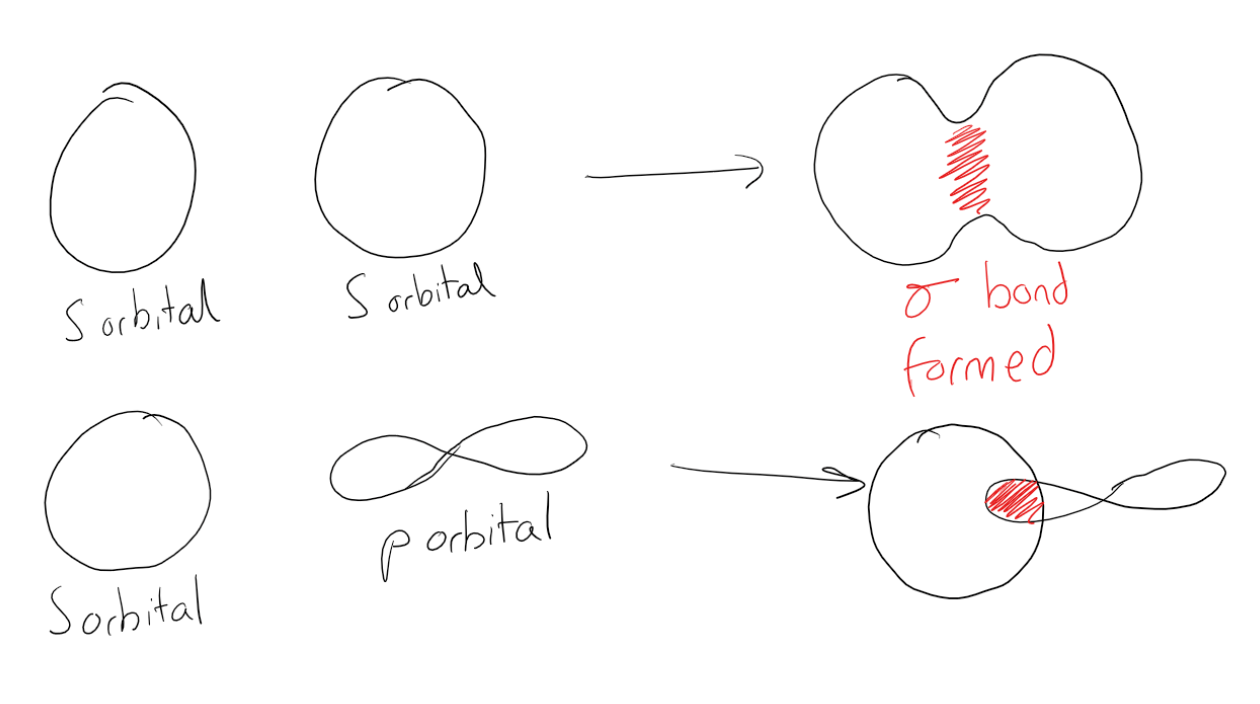Hybrid Orbitals

Double and triple bonds can be explained by orbital hybridization, or the ‘mixing’ of atomic orbitals to form new hybrid orbitals. Hybridization describes the bonding situation from a specific atom’s point of view. A combination of s and p orbitals results in the formation of hybrid orbitals. The newly formed hybrid orbitals all have the same energy and have a specific geometrical arrangement in space that agrees with the observed bonding geometry in molecules. Hybrid orbitals are denoted as spx, where s and p denote the orbitals used for the mixing process, and the value of the superscript x ranges from 1-3, depending on how many p orbitals are required to explain the observed bonding.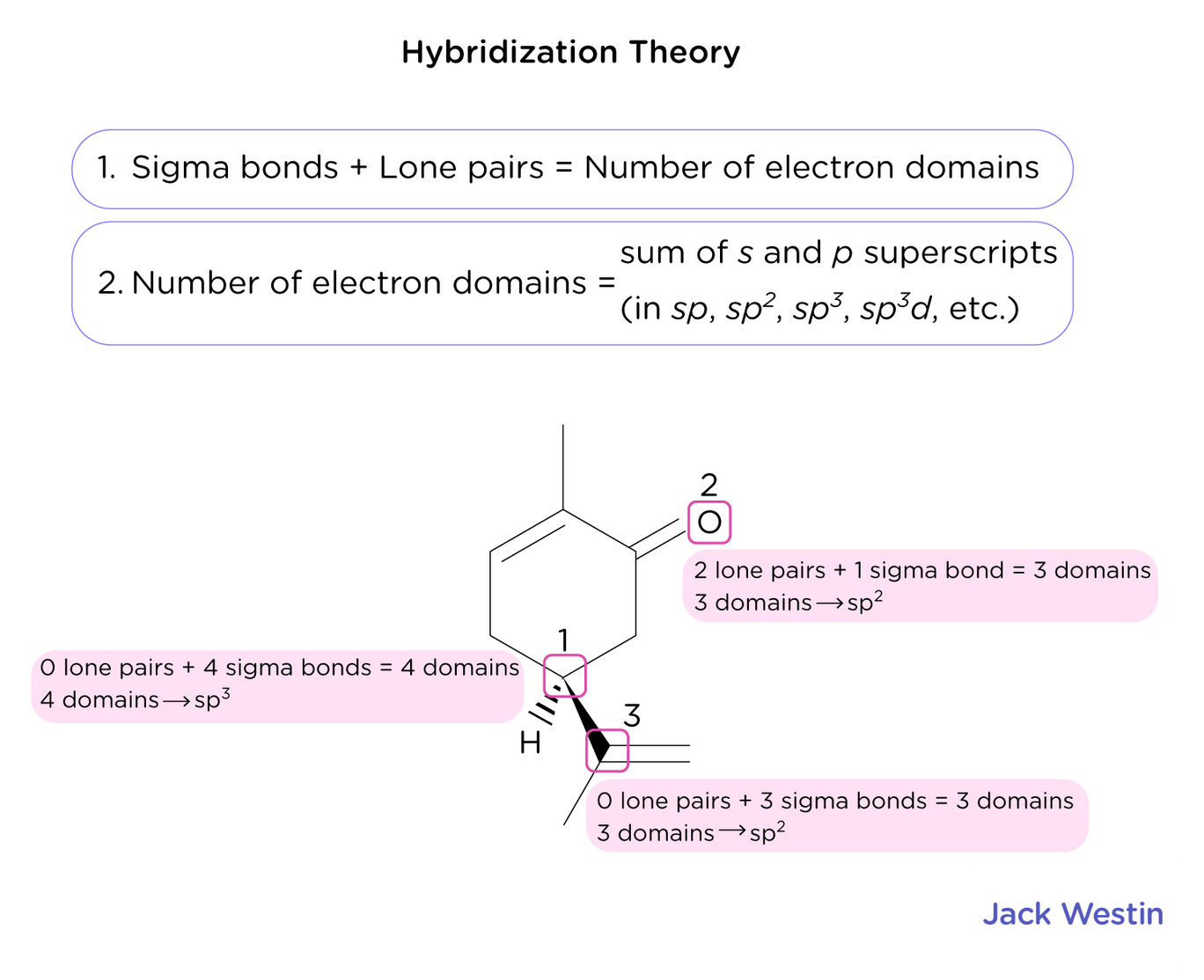Pi or π bonds occur when there is an overlap between unhybridized p orbitals of two adjacent atoms. The overlap does not occur between the nuclei of the atoms, and this is the key difference between sigma and pi bonds. The simplest example of an organic compound with a double bond is ethylene, or ethene, C2H4. The double bond between the two carbon atoms consists of a sigma bond and a π bond.VSPER

Using the 3d representation of atomic orbitals and the bonds they form leads to different angles between the bonds. The valence shell electron pair repulsion (VSEPR) model predicts the shape of individual molecules based on the extent of electron-pair electrostatic repulsion. According to VSEPR, the valence electron pairs surrounding an atom mutually repel each other; they adopt an arrangement that minimizes this repulsion, thus determining the molecular geometry. This means that the bonding (and non-bonding) electrons will repel each other as far away as geometrically possible. The number of bonded pairs of electrons and non bonded pairs of electrons around an atom leads to different geometries: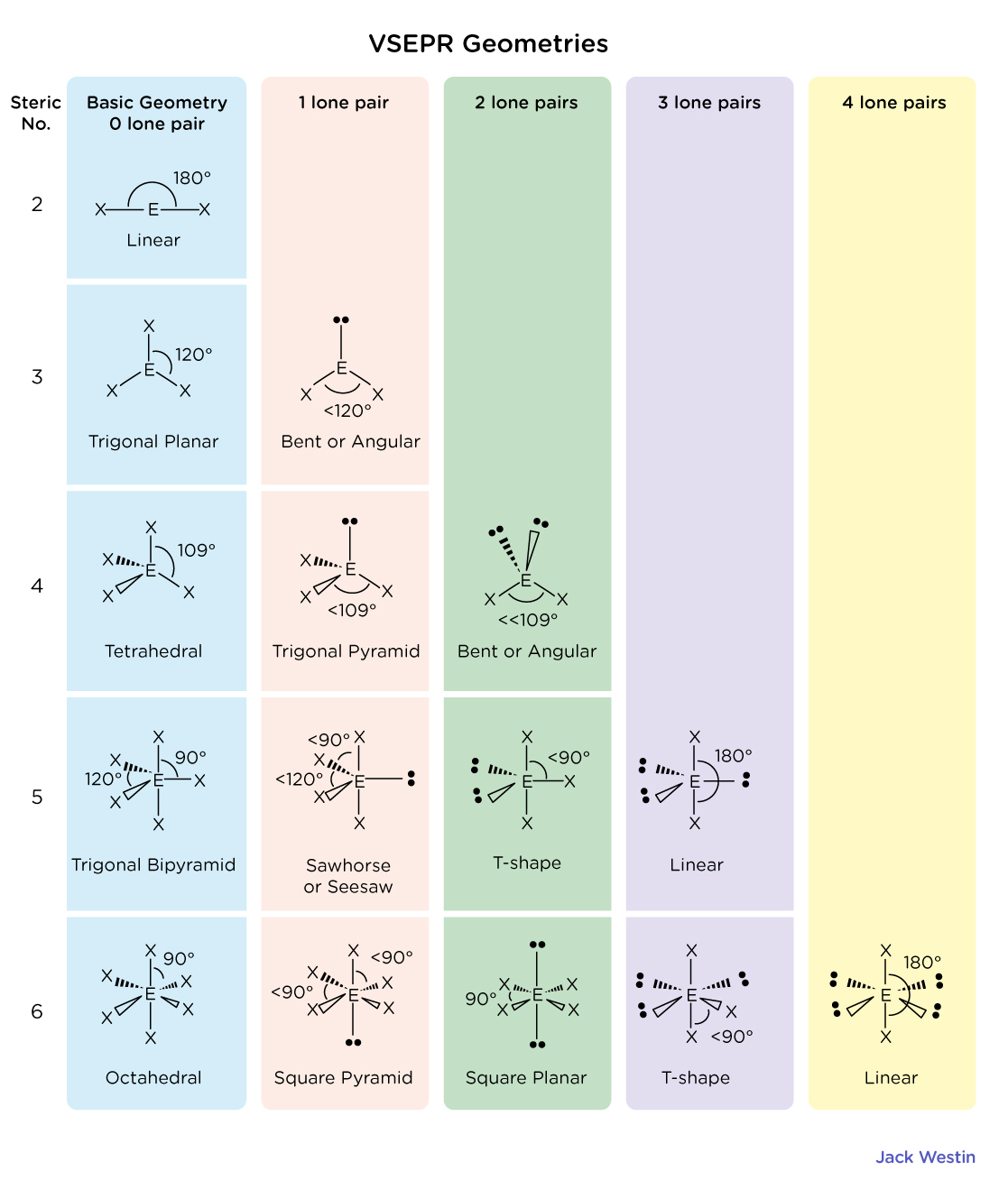The VSEPR theory describes five main shapes of simple molecules: linear, trigonal planar, tetrahedral, trigonal bipyramidal, and octahedral.

• Linear: A triatomic molecule of the type AX2 that has its two bonding orbitals 180° apart, producing a molecule of linear geometry; examples of this include BeCl2 and CO2. Triatomic molecules can also produce different geometries depending on the number of valence electrons.
• Trigonal: In an AX3 molecule, such as BF3, three regions of electron density extend out from the central atom. The repulsion between these will be at a minimum when the angle between any two is 120°.
• Tetrahedral: In an AX4 molecule such as methane (CH4), a central atom is bonded to four other atoms. The four equivalent bonds point in four geometrically equivalent directions in three dimensions corresponding to the four corners of a tetrahedron; this is called tetrahedral coordination. The angle between any two bonds will be 109.5°.
• Octahedral: In an AX6 molecule, six electron pairs will try to point toward the corners of an octahedron (two square-based pyramids joined base-to-base). All the atoms are geometrically equivalent with bond angles of 90°.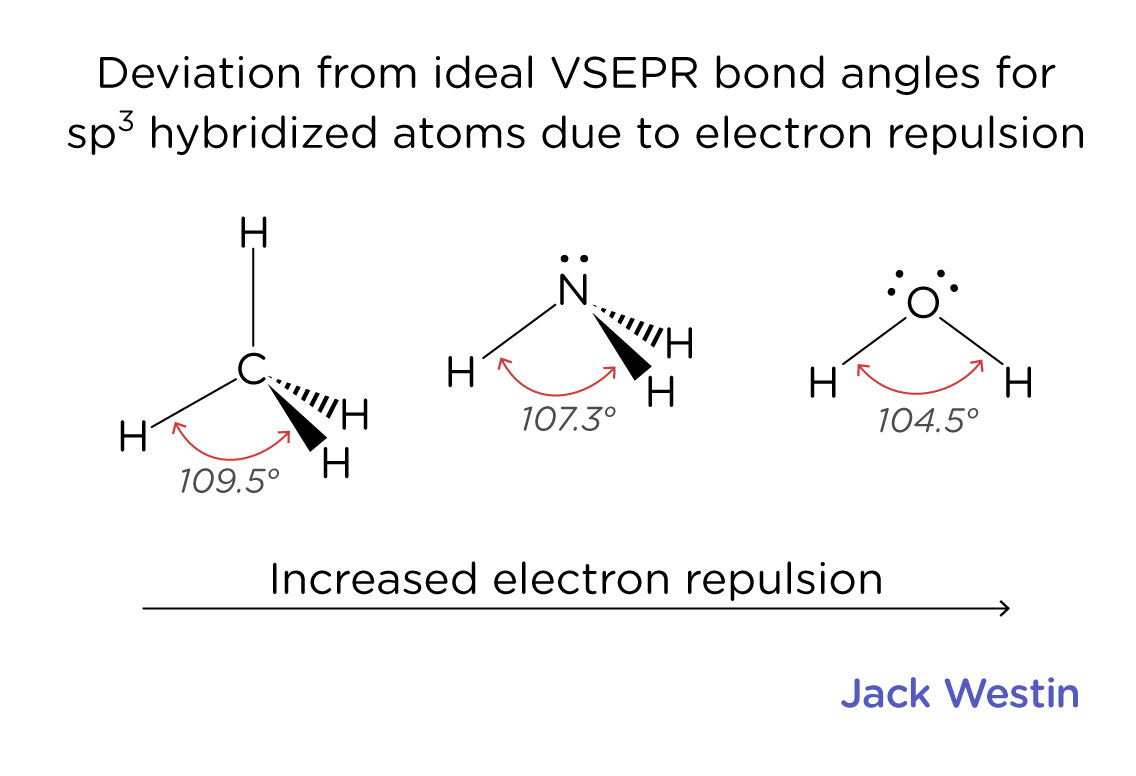Structural formulas for molecules involving H, C, N, O, F, S, P, Si, Cl

Elements in the same column (group) of the periodic table are similar to one another resulting in similar structural formulas: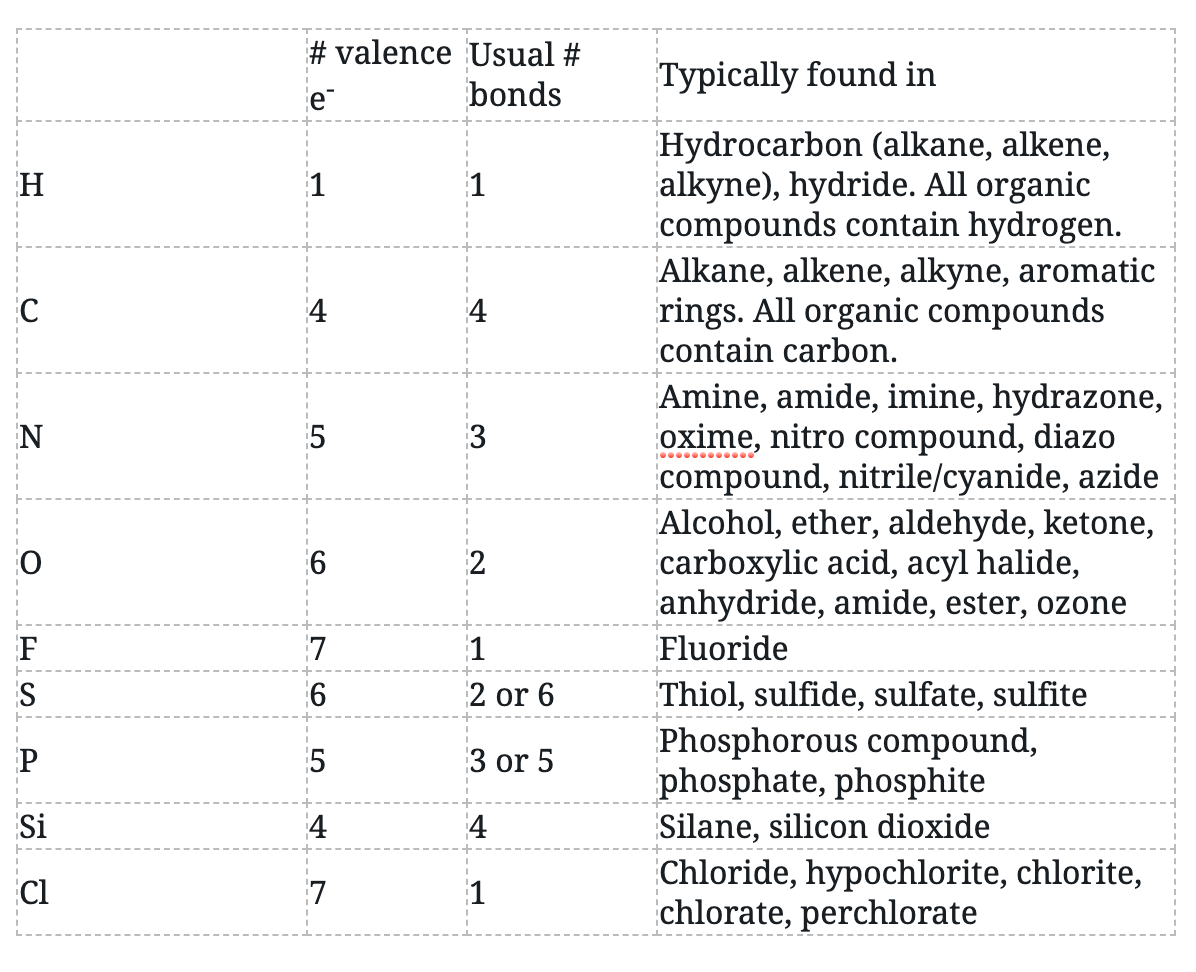Delocalized Electrons and Resonance in Ions and Molecules

Resonance is a mental exercise and method within the Valence Bond Theory of bonding that describes the delocalization of electrons within molecules. It compares and contrasts two or more possible Lewis structures that can represent a particular molecule. Resonance structures are used when one Lewis structure for a single molecule cannot fully describe the bonding that takes place between neighboring atoms relative to the empirical data for the actual bond lengths between those atoms. The net sum of valid resonance structures is defined as a resonance hybrid, which represents the overall delocalization of electrons within the molecule. A molecule that has several resonance structures is more stable than one with fewer. Some resonance structures are more favorable than others.

Electrons have no fixed position in atoms, compounds, and molecules, but have probabilities of being found in certain spaces (orbitals). Resonance forms illustrate areas of higher probabilities (electron densities). The term Resonance is applied when there are two or more possibilities available. Resonance structures do not change the relative positions of the atoms. The skeleton of the Lewis Structure remains the same, only the electron locations change.

In resonance structures, the electrons are able to move to help stabilize the molecule. This movement of the electrons is called delocalization.

1. Resonance structures should have the same number of electrons, do not add or subtract any electrons. (check the number of electrons by simply counting them).
2. All resonance structures must follow the rules of writing Lewis Structures.
3. The hybridization of the structure must stay the same.
4. The skeleton of the structure can not be changed (only the electrons move).
5. Resonance structures must also have the same amount of lone pairs.

Practice Questions

Purification of caffeine

MCAT Official Prep (AAMC)

Chemistry Online Flashcards Question 12

Chemistry Question Pack Passage 1 Question 3

Chemistry Question Pack Passage 11 Question 63

Chemistry Question Pack Passage 19 Question 108

Section Bank C/P Section Question 27

Section Bank C/P Section Passage 5 Question 40

Practice Exam 1 C/P Section Question 28

Practice Exam 2 C/P Section Passage 1 Question 4

Practice Exam 3 C/P Section Question 57

Practice Exam 4 C/P Section Question 12

Practice Exam 4 C/P Section Passage 7 Question 36

Key Points

• A sigma (σ) bond is a bond formed by the overlap of orbitals in an end-to-end fashion, with the electron density concentrated between the nuclei of the bonding atoms.  A pi bond (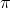bond) is a bond formed by the overlap of orbitals in a side-by-side fashion with the electron density concentrated above and below the plane of the nuclei of the bonding atoms.

• Atomic orbitals S, P, D, and F around an atom can overlap to form sigma bonds.

• These orbitals can hybridize to form larger orbitals with a variety of geometries the most common is the hybridization of the S and P orbitals to form sp1, sp2 and sp3 hybridized orbitals each with its own geometry.

• Pi (π) bonds form from the overlap of unhybridized p orbitals on two adjacent atoms

• VSEPR theory uses the repulsion between bonded pairs and lone pairs of electrons in a molecule to predict the 3d geometry and shape of the molecule.

• Elements in the same column (group) of the periodic table are similar to one another resulting in similar structural formulas.

• Resonance describes the delocalization of electrons within molecules. It compares and contrasts two or more possible Lewis structures that can represent a particular molecule.

Key Terms

Sigma (σ) bond: A covalent bond whose electron density is concentrated in the region directly between the nuclei.

Pi (π) bond: A bond formed by the overlap of orbitals in a side-by-side fashion with the electron density concentrated above and below the plane of the nuclei of the bonding atoms.

Covalent bond: A type of chemical bond where two atoms are connected to each other by the sharing of two or more electrons.

Atomic orbital: A region in space around the atom’s nucleus where there is a probability of finding an electron.

Hybridization: The idea that atomic orbitals fuse to form newly hybridized orbitals, which in turn, influences molecular geometry and bonding properties.

Valence-shell electron pair repulsion (VSEPR): Electron pairs will repel each other such that the shape of the molecule will adjust so that the valence electron-pairs stay as far apart from each other as possible.

Linear: A triatomic molecule of the type AX2 has its two bonding orbitals 180° apart, producing a molecule of linear geometry.

Trigonal: In an AX3 molecule, three regions of electron density extend out from the central atom. The repulsion between these will be at a minimum when the angle between any two is 120°.

Tetrahedral: In an AX4 molecule, a central atom is bonded to four other atoms. The four equivalent bonds point in four geometrically equivalent directions in three dimensions corresponding to the four corners of a tetrahedron. The angle between any two bonds will be 109.5°.

Octahedral: In an AX6 molecule, six electron pairs will try to point toward the corners of an octahedron (two square-based pyramids joined base-to-base). All the atoms are geometrically equivalent with bond angles of 90°.

Resonance: A method of describing the delocalized electrons in some molecules where the bonding cannot be explicitly expressed by a single Lewis structure.

Delocalization: The movement of electrons in resonance structures that help stabilize the molecule.

Billing Information